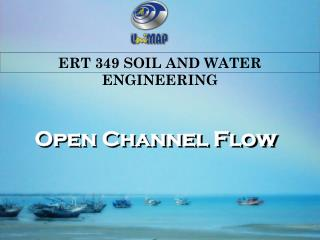DownloadDownload PresentationOpen Channel Flow Part 2

# Open Channel Flow Part 2

Télécharger la présentation## Open Channel Flow Part 2

- - - - - - - - - - - - - - - - - - - - - - - - - - - E N D - - - - - - - - - - - - - - - - - - - - - - - - - - -
##### Presentation Transcript

1. ERT 349 SOIL AND WATER ENGINEERING Open Channel FlowPart 2 Open Channel Flow ERT 349 SOIL AND WATER ENGINEERING Siti Kamariah Md Sa’at PPK Bioproses, UniMAP

2. Channel Design • Open Channel function: • Convey water between 2 points in safe and efficient • Channel Design for uniform flow can be divide to two types: • Lined Channel • Unlined Channel

3. Section factor, Z Conveyance Factor, K • K = AR2/3 /n • From Manning: AR2/3 = Q/So½ n • K = Q/So½ • K is called the conveyance of the channel and express the discharge capacity of the channel per unit longitudinal slope • Z = AR2/3 • From Manning: AR2/3 = Qn/So½

4. Most Efficient Section • Also known as ‘Best Hydraulic Section’ • Characteristics: • Low cost (P min) • Higher Flowrate (Q max)

5. From Manning Equation: With assuming A, n and So is constant, Q max when P min Best hydraulic section has P min Best Hydraulic Section

6. Best Hydraulic Section for Rectangular Channel A = By ------------(i) P =B+2y = A/y + 2y P = A/y + 2y -----------(ii) Best hydraulic section when dP/dy = 0 -Ay-2 + 2 =0 A = 2y2 ---------(iii) y B

7. Best Hydraulic Section for Rectangular Channel From eq (i) A = By And from eq (iii) A = 2y2 B = 2y -----------(iv) R= A/P = 2y2/(B+2y) = 2y2/(2y + 2y) = 2y2/4y = y/2

8. Best Hydraulic Section for Trapezoidal Channel T A = (B + zy)y B = A/y – zy P = B + 2√(z2 + 1) y P = A/y – zy + 2√(z2 + 1) y -------------(v) 1 l y z B

9. Best Hydraulic Section for Trapezoidal Channel dP/dy =-Ay-2 –z + 2√(z2+1) A = (2 √(1 +z2) – z)y2 -------------- (vi) Substitute (vi) in (v) B = 2y(√(1 +z2) – z) P = 2y(2√(1 +z2) – z) R = y/2

10. Best Hydraulic Section for Trapezoidal Channel Side slope, z = 1/√3 And θ = 60o

11. Example 1 • A slightly rough brick-lined trapezoidal channel carrying a discharge of 25 m3 is to have a longitudinal slope of 0.0004. Analyse the proportions of (a) an efficient trapezoidal channel section having a side 1.5 horizontal: 1 vertical (Ans: R=y/2, y=2.830m, B=1.714m) (b) most efficient section of trapezoidal shape (Ans: z =0.57735, y=3.045m,B=3.516m)

12. Example 2 • If z = 1, n = 0.017, So = 1/1200 and Q = 142 m3/min • Determine width of the channel and water depth for most efficient section. • Ans: B = 0.89 m, y = 1.1 m